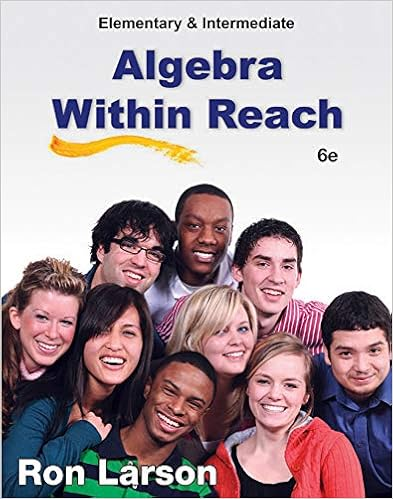# Exam1.950.F18.Review (1).pdf - Math 950 Midterm 1...

• Notes
• ashannescha
• 10

This preview shows page 1 - 3 out of 10 pages.

##### We have textbook solutions for you!
The document you are viewing contains questions related to this textbook.The document you are viewing contains questions related to this textbook.
Chapter 3 / Exercise 74
Elementary and Intermediate Algebra: Algebra Within Reach
LarsonExpert Verified
Math 950 Midterm 1 Objectives Review Please study the following objectives from modules one and two before taking your first midterm. This review provides you with sample problems that correspond to the objectives given, however, the problems on your midterm will not be exactly like the problems on this review.You should go back and study your notes and look at all of the different types of problems that reinforce the objectives below. Module 1– Integers 1.1 Simplify Using the Order of Operations 1.) Simplify: 10 + 4(5 + 2) – 2 2.) Simplify: 16÷ 2 + (6 – 3)21.2 Compare Expressions with Inequality Symbols 3.) Compare the two expressions and fill in with either >, <, or =. –12 –4 4.) Compare the two expressions and fill in with either >, <, or =. –|–6| |–17| 1.3 Add Integers 5.) Simplify:
6.) Simplify: 3 + (–6) + (–8) + 7 1.3 Solve Applications Using Addition of Integers 7.) If you are in a submarine and are at a depth of 70 feet below sea level and rise 53 feet, what depth are you at? 8.) Samantha had \$34 in her bank account. She went to two different stores and spent \$18 at the first store and \$20 at the second store. What is her new balance?
##### We have textbook solutions for you!
The document you are viewing contains questions related to this textbook.The document you are viewing contains questions related to this textbook.
Chapter 3 / Exercise 74
Elementary and Intermediate Algebra: Algebra Within Reach
LarsonExpert Verified
1.4 Subtract Integers 9.) Simplify: –12 – 15 10.) Simplify: –12 – (–15) 1.4 Solve Applications Using Subtraction of Integers 11.) On a Friday in February, the temperature reaches a high of 17° above zero and a low of 2° below zero. What is the difference between the high and low temperatures? 12.) On a cold winter day in Denver, the morning temperature was 4°. By noon it had fallen 15°, and by 5pm it had fallen another 7°. What was the temperature at 5pm? 1.5 Multiply Integers 13.) Simplify: –6(5)(–2) 14.) Simplify: –4(–2 )(–3) 1.5 Divide Integers15.) Simplify: −18616.) Simplify: −7101.5 Simplify Positive Exponents with Integer Bases17.) Simplify: –5218.) Simplify: (–5)21.6 Simplify Using the Order of Operations with Integers 19.) Simplify: 29 – 2(3 – 52) 20.) Simplify: 9 + 20 ÷ (–5) + 2(–3)
•••